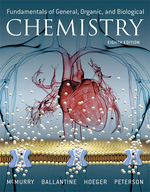×
Get Full Access to Fundamentals Of General, Organic, And Biological Chemistry (Mastering Chemistry) - 8 Edition - Chapter 7.3 - Problem 7.3
Get Full Access to Fundamentals Of General, Organic, And Biological Chemistry (Mastering Chemistry) - 8 Edition - Chapter 7.3 - Problem 7.3

×ISBN: 9780134015187 2044

## Solution for problem 7.3 Chapter 7.3

Fundamentals of General, Organic, and Biological Chemistry (Mastering Chemistry) | 8th Edition

• Textbook Solutions
• 2901 Step-by-step solutions solved by professors and subject experts
• Get 24/7 help from StudySoup virtual teaching assistantsFundamentals of General, Organic, and Biological Chemistry (Mastering Chemistry) | 8th Edition

4 5 1 333 Reviews
20
0
Problem 7.3

In photosynthesis, green plants convert carbon dioxide and water into glucose $$\left(\mathrm{C}_{6} \mathrm{H}_{12} \mathrm{O}_{6}\right)$$ according to the following equation:

$$6 \mathrm{CO}_{2}(g)+6 \mathrm{H}_{2} \mathrm{O}(l) \longrightarrow \mathrm{C}_{6} \mathrm{H}_{12} \mathrm{O}_{6}(a q)+6 \mathrm{O}_{2}(g)$$

(a) Estimate $$\Delta H$$ for the reaction using bond dissociation energies from Table 7.1. Give your answer in kJ/mol. ($$\mathrm{C}_{6} \mathrm{H}_{12} \mathrm{O}_{6}$$. has $$\mathrm{C}-\mathrm{C}$$ bonds, seven $$\mathrm{C}-\mathrm{H}$$ bonds, seven $$\mathrm{C}-\mathrm{O}$$ bonds, and five $$\mathrm{O}-\mathrm{H}$$ bonds).

(b) Is the reaction endothermic or exothermic?

Text Transcription:

C_8/H_12/O_6

6 CO_2 (g)+6 H_2 O (l) longrightarrow C_6 H_12 O_6 (a q)+6 O_2 (g)

C_6H_12O_6

C-C

C-H

C-O

O-H

Step-by-Step Solution:
Step 1 of 3

Friday, September 16, 2016 Week 3 Notes CHE 152 - Ions and the periodic table for main group elements: • - metals lose electrons (until they have the same number of electrons as the nearest noble gas) and become cations - nonmetals tend to gain electrons (until they have the same number of electrons as the nearest noble gas) and become anions • an atomic mass unit (amu) is exactly 1/12 the mass of a carbon-12 isotope • isotopic mass: the mass of a given isotope - the mass number =/= the mass on the periodic table (atomic mass) this is because the atomic mass is the weighted average of the isotopes of a • given element. found by taking the sum of isotopic masses multiplies by their abundance fraction • molar mass: the atomic mass expressed in grams - Avogadro’s number and the mole • Avogadro’s number is 6.022 x 10 23 • the mole is a unit for expressing the number of expressing the number of atoms, ions, molecules, etc. in a “common-size” unit • a mole is the amount of anything that contains Avogadro’s number of units - mole = (number of things) / (6.022 x 10 )23 • the relationship between mass and moles - mole = (mass of the substance) / (molar mass) • compounds have different properties than reactants - there are 119 million known compounds • there are microscale and macroscale equations 1 Frid

Step 2 of 3

Step 3 of 3

## Discover and learn what students are asking

Calculus: Early Transcendental Functions : Iterated Integrals and Area in the Plane
?In Exercises 1 - 10, evaluate the integral. $$\int_{0}^{\cos y} y d x$$

Unlock Textbook Solution